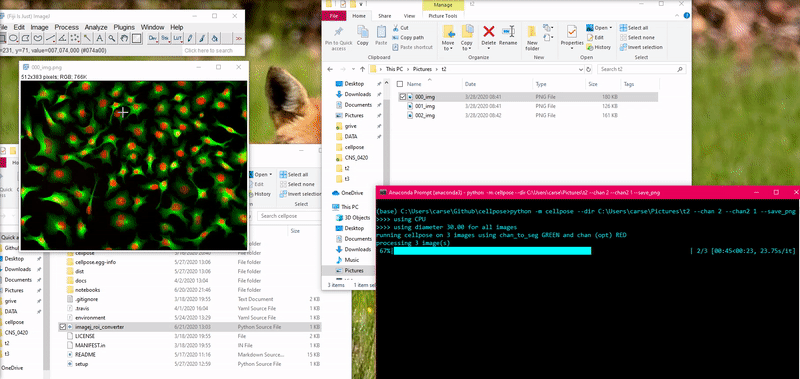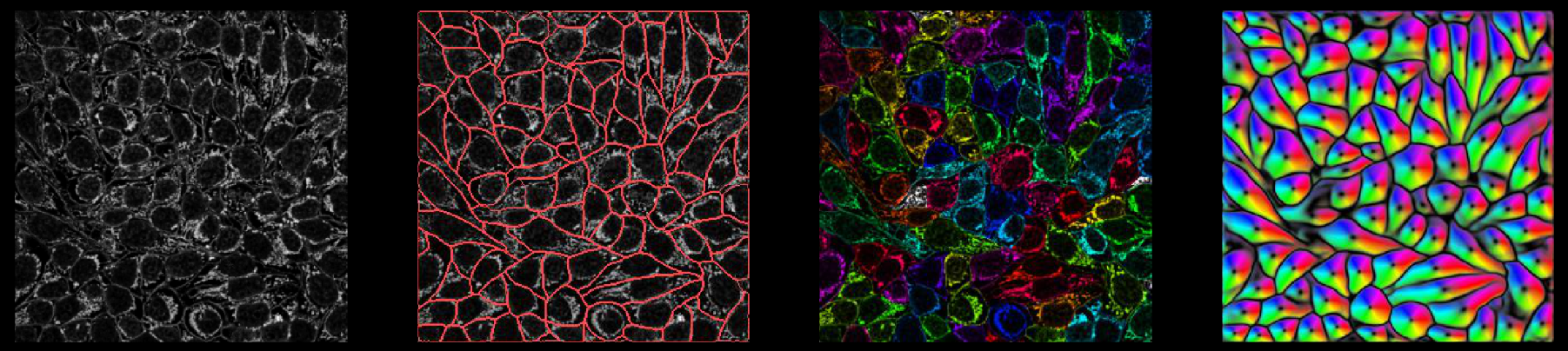# Outputs

Internally, the network predicts 3 (or 4) outputs: (flows in Z), flows in Y, flows in X, and cellprob. The predictions the network makes of cellprob are the inputs to a sigmoid centered at zero (1 / (1 + e^-x)), so they vary from around -6 to +6.

## _seg.npy output

`*_seg.npy` files have the following fields:

• filename : filename of image

• img : image with chosen channels (nchan x Ly x Lx) (if not multiplane)

• masks : each pixel in the image is assigned to an ROI (0 = NO ROI; 1,2,… = ROI labels)

• outlines : outlines of ROIs (0 = NO outline; 1,2,… = outline labels)

• chan_choose : channels that you chose in GUI (0=gray/none, 1=red, 2=green, 3=blue)

• ismanual : element k = whether or not mask k was manually drawn or computed by the cellpose algorithm

• flowsflows is XY flow in RGB, flows is the cell probability in range 0-255 instead of 0.0 to 1.0, flows is Z flow in range 0-255 (if it exists, otherwise zeros),

flows is [dY, dX, cellprob] (or [dZ, dY, dX, cellprob] for 3D), flows is pixel destinations (for internal use)

• est_diam : estimated diameter (if run on command line)

• zdraw : for each mask, which planes were manually labelled (planes in between manually drawn have interpolated ROIs)

Here is an example of loading in a `*_seg.npy` file and plotting masks and outlines

```import numpy as np
from cellpose import plot, utils

# plot image with masks overlaid
colors=np.array(dat['colors']))

# plot image with outlines overlaid in red
plt.imshow(dat['img'])
for o in outlines:
plt.plot(o[:,0], o[:,1], color='r')
```

If you run in a notebook and want to save to a *_seg.npy file, run

```from cellpose import io
```

where each of these inputs is a list (as the output of model.eval is)

## PNG output

You can save masks to PNG in the GUI.

To save masks (and other plots in PNG) using the command line, add the flag `--save_png`.

Or use the function below if running in a notebook

```from cellpose import io
```

## Native ImageJ ROI archive output

You can save the outlines of the ROIs in a ImageJ-native ROI archive file. Rather than using the legacy solution below, you can use this function to create an ROI archive file that can be opened in directly in ImageJ. Recent versions of ImageJ can autodetect the file format. Open in ImageJ using File > Open… and select the file. The ROIs will appear in the ROI manager.

To save the outlines using the CLI use the flag `--save_rois`.

To save the outlines using the API use the `save_rois` function in `io.py`:

This function is also available in the GUI.

```from cellpose import io, utils

# image_name is file name of image

# the file will be saved as '<your_filename_string>_rois.zip'
```

## (Legacy ImageJ Interface) ROI manager compatible output for ImageJ

You can save the outlines of ROIs in a text file that’s compatible with ImageJ ROI Manager in the GUI File menu.

To save using the command line, add the flag `--save_outlines`.

Or use the function below if running in a notebook

```from cellpose import io, utils

# image_name is file name of image
base = os.path.splitext(image_name)
io.outlines_to_text(base, outlines)
```

To load this `_cp_outlines.txt` file into ImageJ, use the python script provided in cellpose: `imagej_roi_converter.py`. Run this as a macro after opening your image file. It will ask you to input the path to the `_cp_outlines.txt` file. Input that and the ROIs will appear in the ROI manager.## Plotting functions

In `plot.py` there are functions, like `show_segmentation`:

```from cellpose import plot

nimg = len(imgs)
for idx in range(nimg):